# New to Qlik Sense

If you’re new to Qlik Sense, start with this Discussion Board and get up-to-speed quickly.

Announcements
QlikWorld online is next week! REGISTER NOW
cancel
Showing results for
Did you mean:Creator

## Calculated dimensions as set modifiers

Hello

I hope I'll be able to explain what I want to achieve, so here it goes:

1. database fields: reporting_date, client, numerical_attribute, sales

2. calculated dimension: for any 2 selected dates, if the numerical attribute of the client has increased, I define a new category named, let's say, "UP", if it has decreased then its "DOWN", stayed the same is "NO CHG"

since I want to be able to calculated this dimension for any 2 reporting dates upon making a selection, I use in the dimension as set modifiers 2 variables based on the reporting date selected (one variable calculates the largest date and the other the smaller date)

so basically my dimension calculates, for any 2 selected reporting dates and for each client, whether the numerical attribute has increased or decreased and assigns to the client either an UP or a DOWN or a NO CHG

I cannot implement this in the script because whether the client is included in UP/DOWN/NO CHG depends on what 2 dates are compared, i.e. the same client can be in eg UP when 2 dates are selected and it can be in eg DOWN when another 2 dates are selected

3. what I want to do is calculate total sales (or increase in sales, it doesn't matter) for the clients which, for 2 dates, are in the UP category and only in this category (or in the DOWN etc, but only for that category)

if the dimension I calculate was a field, I would use a normal modifier sum({<dimension={'UP'}>}sales)

but if instead of "dimension" I write the formula for the dimension, it does not work

if I put the calculated dimension in a table, it works, but I need the value only for each category at a time (for a waterfall chart)

1 Solution

Accepted SolutionsPartner

Example (per analogy)

Hope it would help u :

Let's assume I have a bar chart as follow:

Dimension : Month

Measure: sum(Sales)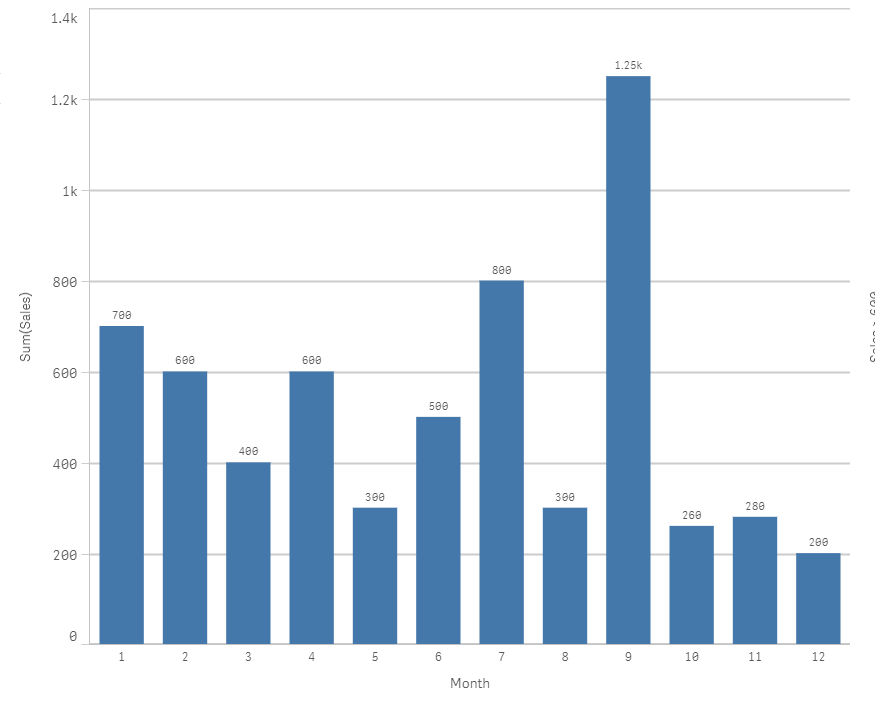As u can see, there is Sales >600 and others <600; what I want to do is create a new dimension which will have 2 values : Sales > 600 and Sales < 600

=> Let's create this calculated dimension :

=aggr(if(sum(Sales)>600,'Sales >600','Sales <600'),Month)So when I click on Sales >600; I 'll only keep the Month that Have sales > 600 :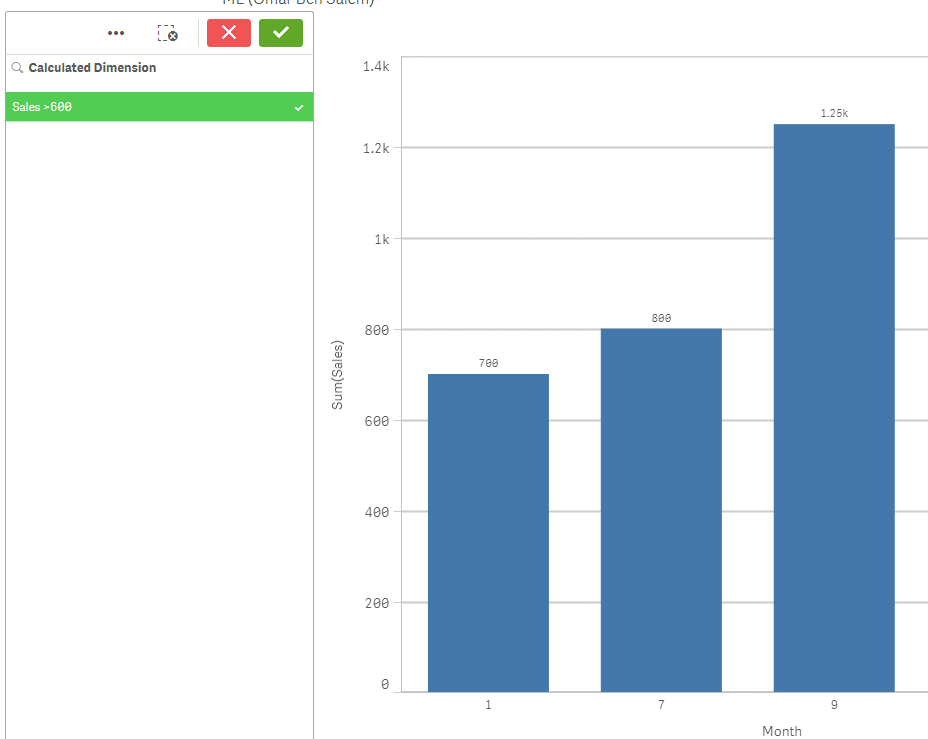Now, what if I wanted to write this directly in the expression of the bar chart; and introduce this newly calculated value Sales > 600 in the set analysis..

Well we can't do it.. BUT we can reconstruct it like follow:

In the bar chart: change the measure

From

sum(Sales)

to

Sum({<Month={"=sum(Sales)>600"}>}Sales)

Result( without any selection):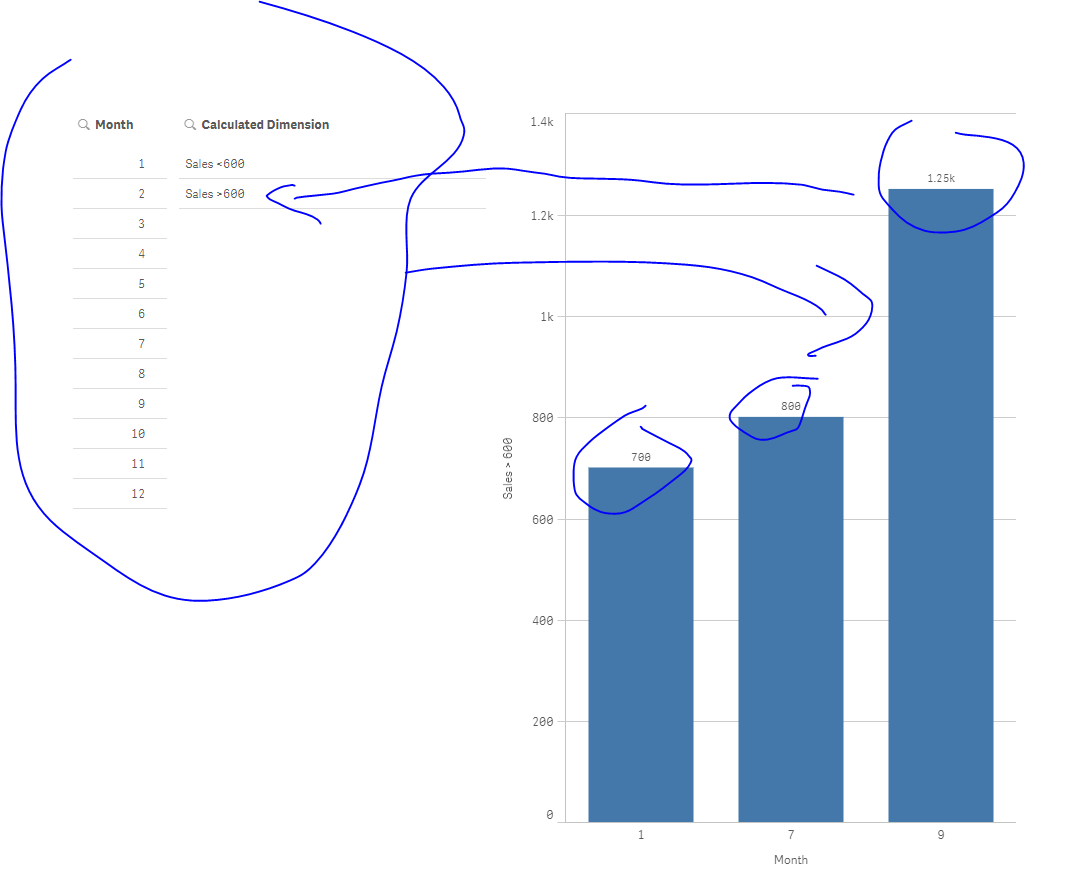Hope u can replcate this logic and it could thus help u!

Omar BEN SALEM

12 RepliesPartner

Can u give an example on how u calculate ur new dimension?Creator
Author

this is the approximate formula (not sure if the syntax is 100% correct because my original formula is more complicated because some clients are new or missing so I need to treat those cases as well etc)

=aggr(if(avg({<reporting_date={'\$(=vMaxDat)'}>}numerical_attribute)-avg({<reporting_date={'\$(=vMinDat)'}>}numerical_attribute)=0, 'NO CHG', if(avg({<reporting_date={'\$(=vMaxDat)'}>}numerical_attribute)-avg({<reporting_date={'\$(=vMinDat)'}>}numerical_attribute)>0,'UP','DOWN')),client)

vMaxDat and vMinDat are the variables that calculate the largest and the smallest date of the 2 dates selectedPartner

Example (per analogy)

Hope it would help u :

Let's assume I have a bar chart as follow:

Dimension : Month

Measure: sum(Sales)As u can see, there is Sales >600 and others <600; what I want to do is create a new dimension which will have 2 values : Sales > 600 and Sales < 600

=> Let's create this calculated dimension :

=aggr(if(sum(Sales)>600,'Sales >600','Sales <600'),Month)So when I click on Sales >600; I 'll only keep the Month that Have sales > 600 :Now, what if I wanted to write this directly in the expression of the bar chart; and introduce this newly calculated value Sales > 600 in the set analysis..

Well we can't do it.. BUT we can reconstruct it like follow:

In the bar chart: change the measure

From

sum(Sales)

to

Sum({<Month={"=sum(Sales)>600"}>}Sales)

Result( without any selection):Hope u can replcate this logic and it could thus help u!

Omar BEN SALEMCreator
Author

it absolutely worked ! (after some trials and tweaks)

OMG Qlik is awesome!

thank you very much for your help!Partner

I confirm; Qlik is awesome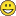ps: my pleasure.Creator
Author

what if in your example I would create 3 categories instead of 2 (let's say 300, 600 and above 600) and I wanted to calculate for 2 of them, let's say < 300 AND 300<X<600 ?

what would this bit <Month={"=sum(Sales)>600"}> look like?

hope I'm not pushing it)Partner

Not at all : sales >=600 and <=800

Sum({<Month={"=sum(Sales)>=600 and sum(Sales)<=800"}>}Sales)

result: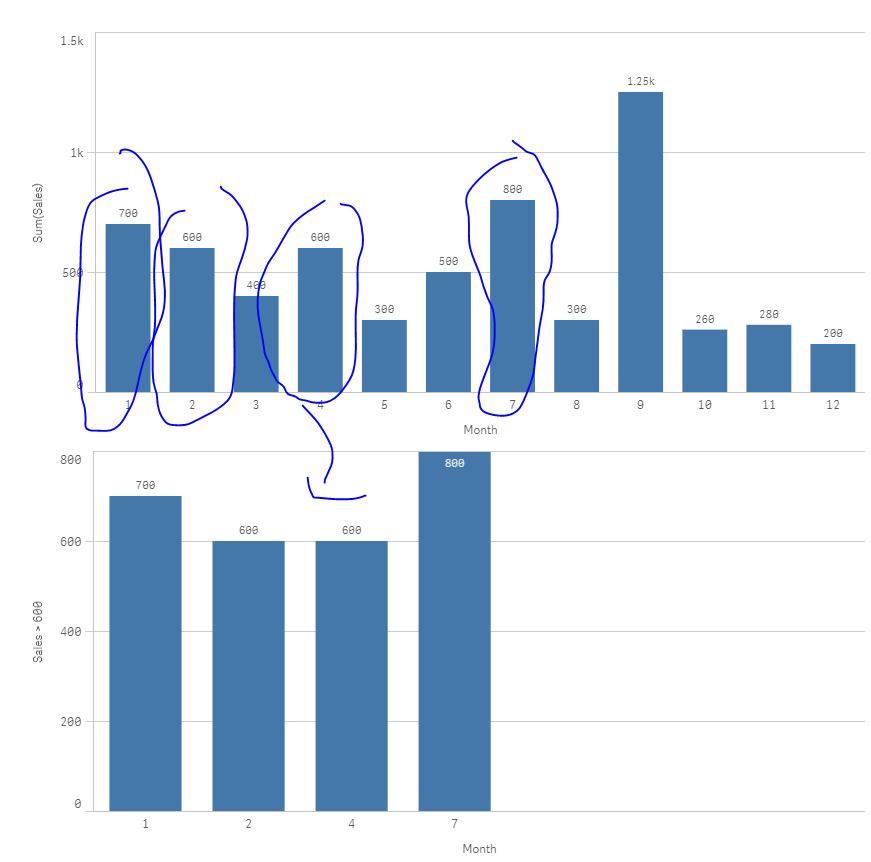Creator
Author

thank youCreator
Author

I have one more question today:

since the formulas can become very long, I thought of using a variable to calculate my dimension

the problem is that I do not know what is the syntax when the calculated dimension is a variable

more specifically, how would this

Month={"=sum(Sales)>600"}

look if sum(Sales) was a variable named, let's say vSales?

I have tried Month={"=\$(=vSales)>600"}

and variations of the like but cannot get it to work

thank you